# C054 – Liquid Totalisation with Correction for Sediment and Water

## Description

This calculates totals whilst correcting for the sediment and water content in the oil.

API Manual of Petroleum Measurement Standards – Chapter 12 – Calculation of Petroleum Quantities – Section 2 – Calculation of Petroleum Quantities Using Dynamic Measurement Methods and Volumetric Correction Factors

IP Petroleum Measurement Manual Part Ⅻ – Static and Dynamic Measurement of Light Hydrocarbon Liquids – Section 1: Calculation Procedures

Kelton calculation reference C054

KIMS calculation reference K137

## Options

### Method

• Injected frequency
• Injected pulses

This option is used to select whether the total will be calculated from the flow rate if frequency is chosen or calculated directly using the number of pulses measured.

### Factor Type

• Meter factor
• K-factor

This option is used to select the whether a meter factor will be applied to the flow rate calculation. Note that K-factor will always be an input.

### Ctsm

• Not applied
• User entered
• Calculated

This option is used to select whether a correction for temperature on the steel of the meter is made and whether this correction is user entered or calculated.

### Cpsm

• Not applied
• User entered
• Calculated

This option is used to select whether a correction for pressure on the steel of the meter is made and whether this correction is user entered or calculated.

### Spool Temperature Coefficient

• Cubic
• Linear (Approximate)
• Linear (Full)

This option is used to select the temperature coefficient applied for the Ctsm calculation is linear or cubic.

### Interpolation Factor

• Apply

This option is used to apply an interpolation factor.

### Calibration Correction

• Apply

This option is used to apply a calibration correction using C244.

### Gross Totals

• Mass
• Observed Volume
• Standard Volume

This option is used to select which gross totals will be calculated.

### Net Totals

• Standard Volume

This option is selected to calculate net standard volume.

### S&W Totals

• S&W Standard Volume

This option is used to calculate the volume of sediment and water.

### Totals

• Use start and end totals

This option allows the user to enter displayed totals for the start and end of the totalisation process for comparison with the calculated results.

### Enter elapsed time as

• Duration
• Start and end time

This option is used to select whether the time over which the totals are to be calculated is entered as a duration or as a start and end time.

### Line density

• User entered
• Calculated

This option is used to select whether line density is user entered or calculated if it is needed.

### Standard density

• User entered
• Calculated

This option is used to select whether standard density is user entered or calculated if it is needed.

## Calculation

### Correction for Sediment and Water

The correction factor for sediment and water is calculated by: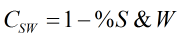Where %S&W = percentage sediment and water content

### Gross Observed Volume

The gross observed volume is calculated by:

If injected pulses is selected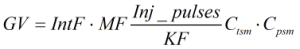If injected frequency is selected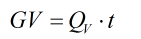Where IntF = Interpolation factor MF = Meter factor Inj_pulses = Injected pulses KF = K-factor Ctsm = Correction for temperature on steel of meter Cpsm = Correction for pressure on steel of meter Qv = Gross observed volume flow rate t = Duration

### Gross Standard Volume

The gross standard volume is calculated by: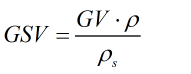Where ρ = Line density ρs = Standard density

### Net Standard Volume

The net standard volume is calculated by: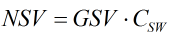### Sediment and Water Standard Volume

The sediment and water standard volume is calculated by: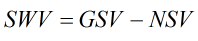Back